## Cipher## Dec 26, 2016

### George Michael - Christmas murder by numbersDied on Christmas at his home in Goring, Oxfordshire
47th prime number is 211 (Pop - 47, Radio - 47, Time - 47, Foundation - 47)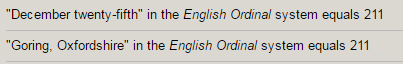12/25/2016 - 12+25+2+0+1+6 = 46 (Sacrifice = 46 E. Reduction)
12/25/2016 - 12+25+20+16 = 73 (Sacrifice = 73)
12/25/16 = 12+25+16 = 53 (Died at the age of 53)

Picture of him in purple stands out, it might be also a connection to Queen Elizabeth coming up later in the post, Purple = 88, Elizabeth = 88His full name is Georgios Kyriacos Panayiotou..... supposedly, but he goes by the name "Michael" = 4+9+3+8+1+5+3 = 33

"Wham! star George Michael has died" was the first title of CNN's postCNN has given us once again unbelievable title, which hints to this story being connected to the Queen Elizabeth who "missed church service today due to heavy cold" but first I have to mention Wham!

The song "Last Christmas" came out on 12/3/1984 = 12+3+19+84 = 118

There's 2 versions of the song and the one that stands out is 6:45 long, six minutes and 45 seconds is 405 seconds,  just like title and band name
So CNN says some interesting stuff in their post

Last Christmas = 3+1+10+2+3+8+9+9+10+2+4+1+10 = 72 - S exceptions
George Michael = 7+5+6+9+7+5+4+9+3+8+1+5+3 = 72 - English Reduction
They mentioned a song called -  "Careless whisper" = 3+1+9+5+3+5+1+1+5+8+9+1+7+5+9 = 72 E. Reduction

It's funny that they start talking about him being gay so randomly, like it's really important
Also they mention his debut album "Faith"

His last album was published on Pi day - 3/14/2014 = 3+14+20+14 = 51 (Conspiracy = 123/51, also they said that "Faith sold over 10 million copies".. 'Ten million' = 123/51)

Don't forget that "Last Christmas" came out on 12/3/1984 on a date with 118 numerology

From release of his last album (3/14/2014) to the day of his death on Christmas this year is 1017 days, now this is where I wanna start connecting things to the Queen Elizabeth
Which is also 33 months and 11 days

1017 is a lot like 117 and today CNN said that Queen missed church service
This is interesting because George Michael died 117 days before Queen's next birthday on April 21st, 2017
Now what was the tipping point for me is CNN giving a clue with Wham! and Last Christmas
The song was released on 12/3 (Conspiracy = 123) in 1984, from the date it was released to death of George Michael is 11711 daysAs I was looking at 117 I thought of November 7 which would be written 11/7 in the US
11/7 is 311th day in the year leaving 54 days, the next date is 11/8 - (Death = 118 in Jewish gematria) - The song "Last Christmas" was released on a date with 118 numerology

November 8th would leave 53 days in the year (he died on a date with 53 numerology at the age of 53)
If we would go to 11/8th and measure to the day of his death we find 1 month and 17 days -Which is 47 days (Time - 47, Pop = 47, Radio = 47 Foundation = 47, Authority = 47...)They like to change titles of the post every 2-4 hours it seems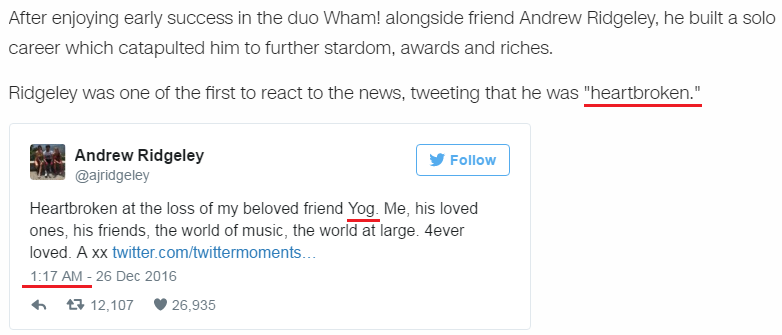Yog - Yours only George - Yog = 47 also Yog is a reflection of Goy

Saturnalia = 44 (S exception), Kill = 44, Saturnalia is old Roman holiday that is today celebrated as Christmas
Saturnian = 117 and 45 with S exception (Magic square of Saturn sums to 45, they say that Saturn is 4.5 billion years old

I would say that this is a sacrifice for the royal family

Also I like how Wikipedia is always updated and ready in the first second you can hear any news about someones death
They say that he died "before 17:24", which is interesting - 17*60 +24 = 1044 ( 144 - 'Forty Four', number connected to killing and all assassinations)

Also he died in a month with astrological sign "Sagittarius" = 144, Killer = 144 (Jewish gematria)
Born June 25th in astrological sign of  "Cancer" - 3+1+14+3+5+18 = 44

Another one of CNN updates, I already noted over 10 title changes over 24 hours
Wham! was on a label called - 'Epic Records' also "Epic" = 5+16+9+3 = 33
From his birthday this year on June 25th to his death is 6 months and 1 day, also to his next birthday from today would be 6 months and 1 day as well

As I'm seeing now they say he died from "Heart failure"

His next album will be coming on 3/3/2017 - 3+3+2+1+7 = 16 - (53, the 16th prime, "Sixteen" = 1+9+6+2+5+5+5 = 33, he died at the age of 53)

Prophecy = 106, also 106 in numerology is seen as 16 the same as release date numerology

## Dec 25, 2016

### AstroNOT Piers Sellers dies at 61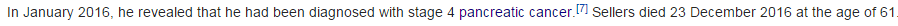Died 11 months after revealing cancer in JanuaryNinety = 5+9+5+5+2+7 = 33
27 in Decimal system is 33 in Octal numbering system

He was born on 4/11/1955 = 4+1+1+1+9+5+5 = 26 (Cancer = 26 E. Reduction), Mason = 26, Lie = 26, God = 26

Died - 12/23/2016 = 1+2+2+3+2+0+1+6 = 17 (Kill - 17, Space - 17)
12/23/2016 = 12+23+2+0+1+6 = 44 (Cancer = 44, Kill = 44, Space = 44) he was born on 4/11 (4x11 = 44)
12/23/2016 = 12+23+20+16 = 71 (Pancreatic cancer = 71)
12/23/16 = 12+23+16 = 51 (Conspiracy = 51, he was chosen for NASA Group 16 on 5/1/1996)

December = 55, he was born in 1955,  he died on 257th day after his birthday this year (55th prime number is 257), Sacrifice = 55 (S exception)On October 21st this year was released a documentary film called "Before the flood"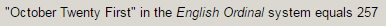October = 6+3+2+6+2+5+9 = 33
Released - 10/21/16 = 10+21+16 = 47 (his middle name "John" = 47, Foundation = 47, Authority = 47, Obey = 47, Time = 47, Agent = 47, Star of David = 47)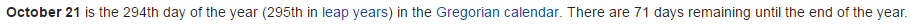October 21st leaves 71 days in the year, Pancreatic cancer = 71, and he died on a date numerology of 71Director of "Earth Science"He died 110 days before his next birthday

## Dec 23, 2016

### Adolf Hitler - SS - Nazi Germany

Starting with Paul von Hidenburg who was President of Germany before HitlerHe was a German Military Officer and 2nd President of Germany
Military = 4+9+3+9+2+1+9+7 = 44, Officer = 6+6+6+9+3+5+9 = 44

He died at the age of 86 from 'lung cancer'Died on August Second, 1934 which is the day when Hitler became Fuhrer of Germany
'August' = 1+3+7+3+1+2 = 17 Kill = 2+9+3+3 = 17 - E. Reduction
'Second' = 10+5+3+6+5+4 = 33 with the S exception

8/2/1934 - 8+2+1+9+3+4 = 27 (died in 'Neudeck' = 5+5+3+4+5+3+1 = 27)
8/2/34 - 8+2+34 - 44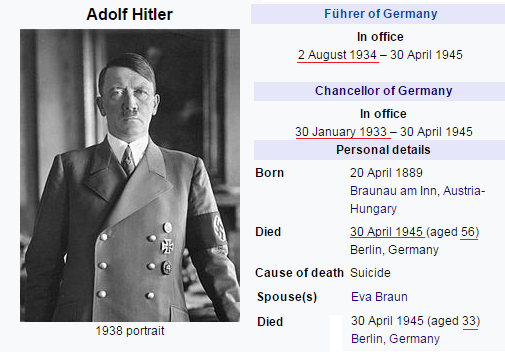Born on April 20th in 1889 which is 110th day in the yearPresident = 7+9+5+10+9+4+5+5+2 = 56 with the S exception just like Adolf Hitler

The date of his birth can also be written like this - 4/20/89 - 4+20+89 = 113
He became Chancellor of Germany on 1/30/33 = 64 (64th prime number is 113)

1/30/1933 - 1+30+1+9+3+3 = 47 (Authority = 47, Foundation = 47, Obey = 47, Framework = 47, Star of David = 47)

From his 43rd birthday in1932 to January 30th 1933, the day when he became the Chancellor is 9 months 11 days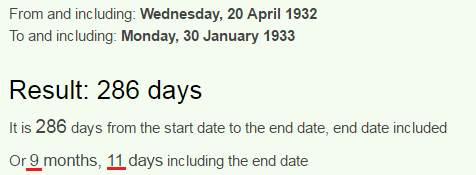Also from January 30th to his 44th birthday is 2 months and 22 days, which is also 11 weeks and 4 days (His birthday in 1933 has 22 numerology as well - 4/20/1933 = 4+2+0+1+9+3+3 = 22)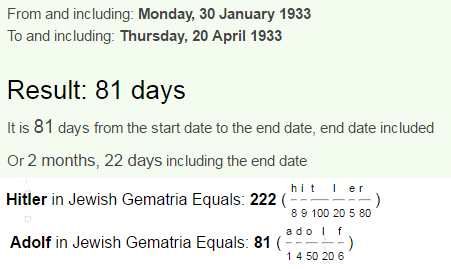Nazis = 33, Holocaust = 33, Berlin = 33, Zyklon B = 33 (Gas used to kill people in concentration camps)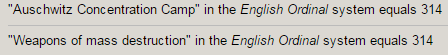And from his birthday in 1934 to the day when The 2nd President of Germany died and he became Fuhrer is 3 months and 14 days  ( 8/2/34 - 8+2+34 = 44)Masonry - 105, Zionism - 105
In numerology 105 is seen as 15 since 0 doesn't count, 15th prime number is 47, also 105 days is exactly 15 weeks

From his birthday in 1934 to his death - 4/30/1945 is exactly 11 years and 11 moths...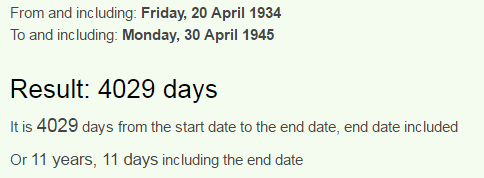Moving on from measuring days for nowAlso known as Nazi Party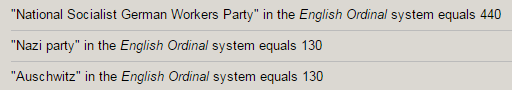Hitler was chairman of Nazi party for almost entirety of it's existence

It was founded on February 24th in 1920 - February is the only month that sums to 42, it was established on 24th day a reflection of 42 (Hitler was chairman for 24 years)
February 24th is 55th day in the year
World War Two = 5+6+9+3+4+ 5+1+9 + 2+5+ 6 = 55

2/24/1920 - 2+24+1+9+2+0 = 38 (Germany = 38, Death = 38, Killing = 38, Adolf = 38, Jew = 38, Palestine = 38, Soldiers = 38)

Schutzstaffel  (SS) was a military order created 5 years after establishment of Nazi Party
SS flag can be seen as 44 (Kill = 44, Military = 44, Genocide = 44.. )
If you take it as double SS it can be 38 (Death = 38, Killing = 38, Soldiers = 38, Germany = 38, Adolf = 38)
Formed on April 4th, 1925 (4/4 a lot like 44), April Fourth leaves 271 days in the year (58th prime number is 271 - Freemasonry = 58, Secret Society = 58, Solomon's Temple = 58 Global economy = 58) also the Agency was dissolved on May 8th which can be written as 5/8

Formed - 4/4/25 = 4+4+25 = 33
Dissolved - 5/8/45 = 5+8+45 = 58
5/8/1945 - 5+8+19+45 = 77 (33-77 is very frequent) Judaism = 77, Christ = 77

They also say that SS was officially founded on 11/9/1925

11/9/1925 - 1+1+9+1+9+2+5 = 28 (Twenty-eight = 156, Thirty-three = 156, 156th prime number is 911 a reflection of 119)

119 has a connection to 144, but is a popular number on itself being a reflection of 911 and Star of David = 119, All seeing eye = 119, Foundation = 119, Saturn = 119 (In Francis Bacon system - lower case A-Z is 1-26, upper case A-Z is 27-52)

5.5 million - 6 million Jews is truly hilarious to me
But they can give us even more
44th prime number is 193..

Also I forgot to mention that Hitler's wife Eva Braun was 33 years old at the time they committed suicide